#Function Repository Resource:

# AntidiagonalMatrixQ

Tests whether a matrix is an antidiagonal matrix

Contributed by: Sander Huisman
 ResourceFunction["AntidiagonalMatrixQ"][m] gives True if m is antidiagonal, and False otherwise. ResourceFunction["AntidiagonalMatrixQ"][m,k] gives True if m has nonzero elements only on the kth antidiagonal, and False otherwise.

## Details and Options

An antidiagonal matrix is defined as a matrix whose elements are zero except for its antidiagonal: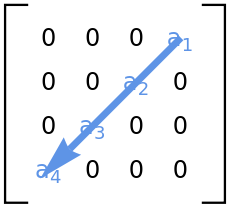ResourceFunction["AntidiagonalMatrixQ"][m] works even if m is not square.
For positive k, ResourceFunction["AntidiagonalMatrixQ"][m,k] tests whether the antidiagonal is above the leading antidiagonal. ResourceFunction["AntidiagonalMatrixQ"][m,-k] tests whether the antidiagonal is below.
ResourceFunction["AntidiagonalMatrixQ"] works on SparseArray matrices.

## Examples

### Basic Examples

Check if a matrix is an antidiagonal matrix:

 In:=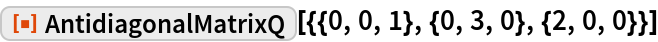Out=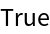Test if the antidiagonal is above the leading antidiagonal one:

 In:=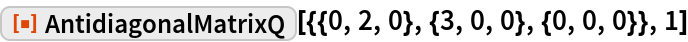Out=An example of a matrix that is not antidiagonal:

 In:=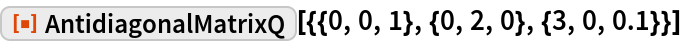Out=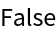### Scope

A complex matrix:

 In:=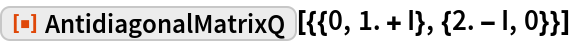Out=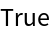Test a rectangular matrix:

 In:=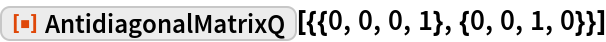Out=Test a SparseArray matrix:

 In:=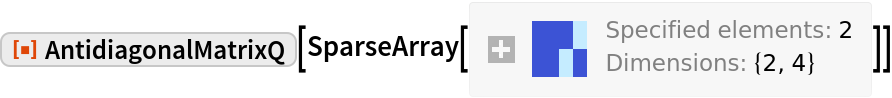Out=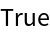### Options

#### Tolerance

Check if the off-antidiagonal elements are zero within a certain tolerance:

 In:=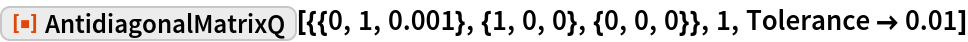Out=### Properties and Relations

If the expression is not a matrix it will return false:

 In:=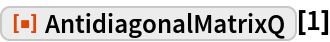Out=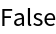In:=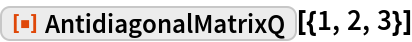Out=